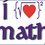# Angle problem!

Please,im dying to know the solution of this problem .Can someone please provide one ,only using elementary geometry if possible,if not then use trig. In the equilateral triangle $ABC$ inside angle $A$ but outside the triangle is given a point M, such that $AMC=30$ and $AMB=40$.Find the angles of triangle $BMC$.Please try to solve it .Note by Lawrence Bush
6 years, 4 months ago

This discussion board is a place to discuss our Daily Challenges and the math and science related to those challenges. Explanations are more than just a solution — they should explain the steps and thinking strategies that you used to obtain the solution. Comments should further the discussion of math and science.

When posting on Brilliant:

• Use the emojis to react to an explanation, whether you're congratulating a job well done , or just really confused .
• Ask specific questions about the challenge or the steps in somebody's explanation. Well-posed questions can add a lot to the discussion, but posting "I don't understand!" doesn't help anyone.
• Try to contribute something new to the discussion, whether it is an extension, generalization or other idea related to the challenge.

MarkdownAppears as
*italics* or _italics_ italics
**bold** or __bold__ bold
- bulleted- list
• bulleted
• list
1. numbered2. list
1. numbered
2. list
Note: you must add a full line of space before and after lists for them to show up correctly
paragraph 1paragraph 2

paragraph 1

paragraph 2

[example link](https://brilliant.org)example link
> This is a quote
This is a quote
    # I indented these lines
# 4 spaces, and now they show
# up as a code block.

print "hello world"
# I indented these lines
# 4 spaces, and now they show
# up as a code block.

print "hello world"
MathAppears as
Remember to wrap math in $$ ... $$ or $ ... $ to ensure proper formatting.
2 \times 3 $2 \times 3$
2^{34} $2^{34}$
a_{i-1} $a_{i-1}$
\frac{2}{3} $\frac{2}{3}$
\sqrt{2} $\sqrt{2}$
\sum_{i=1}^3 $\sum_{i=1}^3$
\sin \theta $\sin \theta$
\boxed{123} $\boxed{123}$

Sort by:

Thank you all,Very much !

- 6 years, 4 months ago

I'm finding it tricky to solve this using just elementary geometry. but using a mess of trig I'm finding that one solution seems to be $\angle BCM = 70^{\circ}$ and $\angle CBM = 40^{\circ}$, (and of course $\angle BMC = 70^{\circ}$ as given). The computed values appear to be exact, so there is definitely hope that there is a solution method using elementary geometry. I'll keep trying to find one, but in the meantime I'm confident that these are the angles you're looking for. The following is my trig approach.

Let $\angle MAC = x$ and without loss of generality let the equilateral triangle have side length $1.$ Also, let $|MC| = a$ and $|BM| = b.$

Using the Sine rule on $\Delta AMC$ we have that $\dfrac{\sin(\angle AMC)}{1} = \dfrac{\sin(x)}{a} \Longrightarrow a = 2\sin(x).$

Using the Sine rule on $\Delta AMB$ we find that $\dfrac{\sin(\angle AMB)}{1} = \dfrac{\sin(60^{\circ} - x)}{b} \Longrightarrow b = \dfrac{\sin(60^{\circ} - x)}{\sin(40^{\circ})}.$

Next, using the Cosine rule on $\Delta BMC$, we have that $1 = a^{2} + b^{2} - 2ab\cos(70^{\circ}).$ Upon substitution of our expressions for $a$ and $b$ into this last equation, we find that

$1 = 4\sin^{2}(x) + \dfrac{\sin^{2}(60^{\circ} - x)}{\sin^{2}(40^{\circ})} - 4\sin(x)\sin(60^{\circ} - x)*\dfrac{\cos(70^{\circ})}{\sin(40^{\circ})}.$

Now this does look like a mess, but notice that for $x = 20^{\circ}$ the RHS of the equation reduces to

$4\sin^{2}(20^{\circ}) + 1 - 4\sin(20^{\circ})\cos(70^{\circ}) = 1$, i.e., $x = 20^{\circ}$ satisfies the equation.

From here we can then quickly determine that $\angle BCM = 70^{\circ}$ and $\angle CBM = 40^{\circ}$, as indicated before. So we got a bit lucky there that the answer was so nice. :)

(Note that I did find a second possible solution to the messy trig equation of approximatley $x = 25.62628^{\circ}$, but I have yet to determine whether or not it is extraneous.)

- 6 years, 4 months ago

Darn! You beat me to posting the solution sir . But I guess no one can beat the Math King :D

- 6 years, 4 months ago

The other solution arises form the case where M and A are on the same side of BC, and so M is inside the "backwards angle" of $\angle BAC$. We would actually have $\sin x = \ldots$, and the solution that we want is $x = 180^ \circ - 25.6 ^ \circ$.

Staff - 6 years, 4 months ago

Here's a simple synthetic geometry approach:

Hint: Prove that $B$ is the circumcenter of triangle $ACM$.
(This can be proven by using 2 obvious facts in the diagram.)

Hence, conclude that $BC = BM$ and thus we have a $70 ^ \circ - 70 ^ \circ - 40 ^ \circ$ triangle.

Thanks to Brian for doing the hard work of finding out that we have an isosceles triangle so $BM = BC = BA$, which motivates proving that $B$ is the circumcenter of the points $A, C, M$.

Staff - 6 years, 4 months ago

In triangle BMC, Angle B = 40, C = 70 and M -= 70. The only point of import is that BM is equal to a side of equilateral triangle BC, as angle AMC = 30 (given) which is half of angle ABC.

- 6 years, 4 months ago

Did you graduate from IIT KGP sir ?

- 6 years, 4 months ago

Yes, 1970 B.Tech. (ECE)

- 6 years, 4 months ago

Cool!

- 6 years, 4 months ago